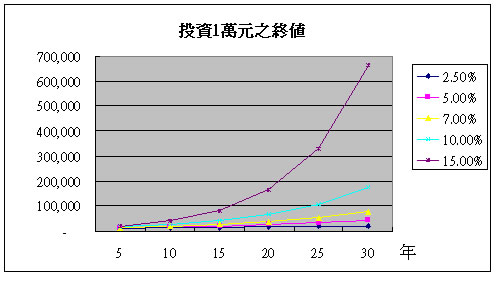﻿

# 唯有理財才可能致富

## 定存也只是聊勝於無

=FV(2.5%, 30, -500000) = 21,951,352

=PV(2.5%, 30, 0, 21951352) = 10,465,146

## 看看會理財的人

=FV(15%, 30, -500000) = 217,372,573

### 考慮通貨膨脹後

=PV(2.5%, 30, 0, 217372573) = 103,630,784

# 複利的威力無窮FV = PV*(1+R)n## 奇妙的72法則

 報酬率 ( R ) 72法則 =72 / (R*100) 精確值 =ln(2) / ln(1+R) 2.5% 28.8 28.1 5.0% 14.4 14.2 7.0% 10.3 10.2 10.0% 7.2 7.3 15.0% 4.8 5.0 20.0% 3.6 3.8

 時間(年) 1元的終值 0 1 5 1*2 = 2 10 2*2 = 4 15 4*2 = 8 20 8*2 = 16 25 16*2 = 32 28.3 52

# 附註：

FV = PV*(1+R)n

FV/PV = (1+R)n

2 = (1+R)n

ln(2) = ln(1+R)n = n*ln(1+R)

n = ln(2) / ln(1+R)

﻿

■ 本網站內容儘可能精確完整，但不保證無誤。若做為投資依據，風險請自行斟酌 ，本網站不負賠償之責任。
■ 網站所有資料均為版權所有，非經書面允許請勿轉載或使用。